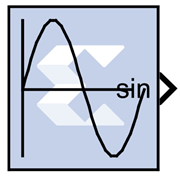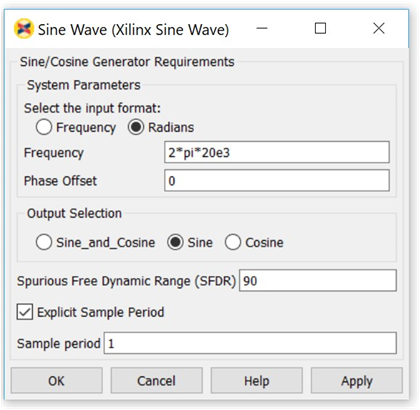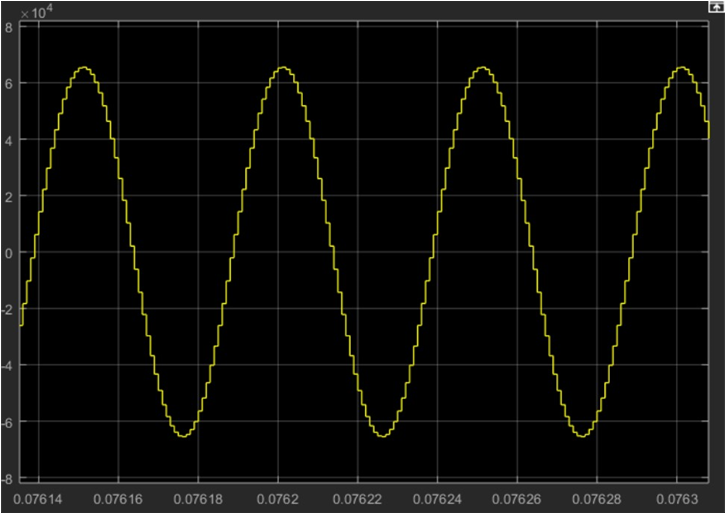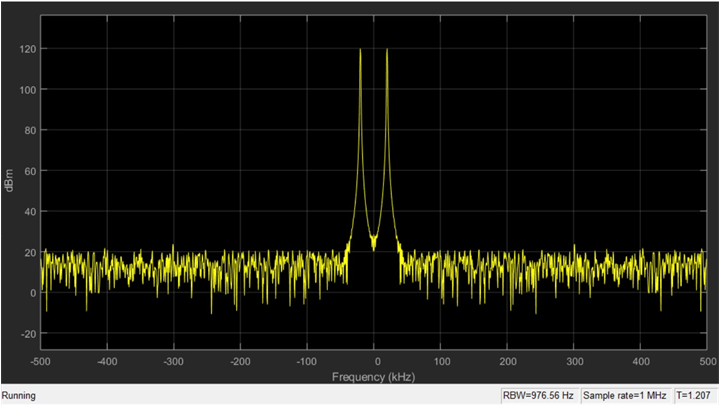# Sine Wave - 2020.2 English

## Vivado Design Suite Reference Guide: Model-Based DSP Design Using System Generator (UG958)

Document ID
UG958
Release Date
2020-11-18
Version
2020.2 English

This block is listed in the following Xilinx® Blockset libraries: DSP and Index.

The Xilinx Sine Wave block generates a sine wave, using simulation time as the time source.The Xilinx Sine Wave block outputs a sinusoidal waveform. Outputs from the block can be a sine wave, a cosine wave, or both. When implemented in a Xilinx FPGA or SoC, the Sine Wave block optimizes the block parameters for your target device.

The output of the Sine Wave block is determined by this equation:

y = sin (2 π (k+o)/p)

where

p = number of time samples per sine wave period

k = repeating integer value that ranges from 0 to p-1

o = offset (phase shift) of the signal

In this block, Xilinx System Generator sets k equal to 0 at the first time step and computes the block output, using the formula above. At the next time step, Simulink increments k and re-computes the output of the block. When k reaches p, Simulink resets k to 0 before computing the block output. This process continues until the end of the simulation.

The output characteristic of the Sine Wave block is determined by:

Samples per period = 2π / (Frequency * Sample Time)

Number of offset samples = Phase Offset * Samples per period / 2π

The Sine Wave block is ideal for generating simple sine and cosine waves. If your sine wave implementation will use more complicated features such as a phase generator, multiple channel support, or AXI4 ports, use the Xilinx DDS Compiler 6.0 block in your design instead of the Sine Wave block.

In the Vivado design flow, the Sine Wave block is inferred as "LogicCore IP DDS Compiler v6.0" for code generation.

## Block Parameters

The block parameters dialog box can be invoked by double-clicking the icon in your Simulink® model.

Parameters specific to the block are as follows:

System Parameters
Select the input format
Specifies whether the frequency and phase offset inputs are entered as a Frequency (Hz) or an angular velocity (Radians) value.
Frequency
Specifies the frequency, either in Hertz or radians. The default is 1.
Phase Offset
Specifies the phase shift, either in Hertz or radians. The default is 0.
Output Selection
Sine_and_Cosine
Places both a sine and cosine output port on the block.
Sine
Places only a sine output port on the block.
Cosine
Places only a cosine output port on the block.
Spurious Free Dynamic Range (SFDR)
Specifies the precision of the output produced by the Sine Wave block. This sets the output width as well as internal bus widths, and controls various implementation decisions.
Explicit Sample Period
If checked, the Sine Wave block uses the explicit sample time specified in the Sample Period box below. If not checked, the System Generator base period will be used as block sample time.
Sample Period
If Explicit Sample Period is selected, specifies the sample time for the block.

## Example

A simple use case of generating sinusoidal signal using Sine Wave block is shown below.

To generate a 20 KHz sine wave with π/2 phase offset in a system running at sample period of (1/1e6) or 1 MHz, use the following specification on the Sine Wave block.

Figure 1. Sine Wave SpecificationsThese settings generate this sine wave:

Figure 2. Sine Wave SettingsWavelength of sine wave = Simulink Sample Period / Frequency => 1MHz/20KHz = 0.5 * 10-4

The spectrum view of the sine wave is:

Figure 3. Sine Wave OutputAlso:

Number of Samples per period = (2π/(1/1e6 * 20e3))

= 50 (Total number of samples in a single cycle)

Number of offset samples = (π/2) * (50/2π) = 50/4